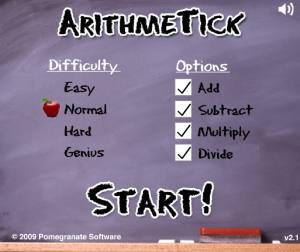## ThanksGiving Day

¿Cuál es el origen del Día de Acción de Gracias?

If you like, you can print these monsters too

I hope you like them.

## Maths in English

Play this game and learn:

Sumar → plus → 3 plus 5 = 8
Restar → minus → 5 minus 2 = 3
Multiplicar → times → 2 times 7 = 14
Dividir → divided by → 10 divided by 2 = 5
Igual → equals